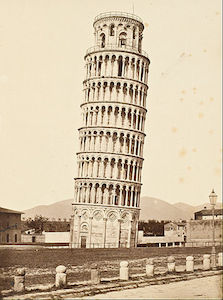Calculus

# Displacement, Velocity, Acceleration Word ProblemsGalileo's famous Leaning Tower of Pisa experiment demonstrated that the time taken for two balls of different masses to hit the ground is independent of its weight. Assuming that the following table indicates the vertical height $h$ that the cannonballs dropped $t$ seconds after being released till it hits the ground and comes to rest.

 time $t$ 0 0.5 1 1.5 2 2.5 3 height $h$ 0 1 5 11 20 30 44

Which of the following is the best approximation for the instantaneous speed at $t = 2.5$?

If (on a particular planet) a stone is thrown straight up from $80$ m above ground at a velocity of $70$ m/s, then the height above the ground $S(t)$ in meters $t$ seconds after it is thrown is $S(t)=80+70t-10t^2.$ What is the velocity of the stone at the instant it lands on the ground?

If the position of a particle at a time $t$ is given by the equation $x(t)=t^3-11t^2+24t,$ what is the acceleration of the particle at time $t=5 ?$

The movie "Snowpiercer" is a story about the survivors on a train in an icy world where everything is frozen because of climate change. The production team is preparing for today's shooting, which could be very dangerous because the train has to make an extremely sudden stop in front of a huge ice cliff right before hitting it. The staff engineers' simulation shows the train will continue to proceed $x=27t-0.45t^2$ meters for t seconds after the stuntman steps on the brake. How far away in meters from the ice cliff must the stuntman step on the brake so that the train can stop as desired?

The area of a circle is increasing at the rate of $54\pi \text{ in}^2\text{/sec}.$ When the radius of the circle is $3 \text{ in},$ what is the rate of increase of the radius?

×